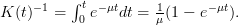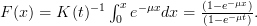Want to share your content on R-bloggers? click here if you have a blog, or here if you don't.

I recently posted the message below with regard to sampling from the truncated exponential distribution. I left out the derivation of the CDF (mostly because text math is ugly), so I’ve included it here. There is also a short JSS article about truncated distributions in R. This problem in particular may likely be found in an introductory text on survival analysis or probability theory.

Where$t$ is the level of truncation and$mu$ is the rate, the normalization constant$K(t)$ is given by$K(t)^{-1} = int_0^t e^{-mu t} dt = frac{1}{mu} (1-e^{-mu t}).$

The truncated exponential CDF is then$F(x) = K(t)^{-1}int_0^x e^{-mu x} dx = frac{(1-e^{-mu x})}{(1-e^{-mu t})}.$

Solving for the inverse CDF yields the itexp function below. From the R-help list:

Since there is a simple closed form for the truncated exponential CDF, you can use inverse transform sampling. I believe this is quite common in survival analysis methods. The first step is to compute and write an R function to compute the inverse CDF for the truncated exponential, say

`itexp <- function(u, m, t) { -log(1-u*(1-exp(-t*m)))/m }`

where u is the quantile, m is the rate, and t is the level of truncation. Next, we draw from the truncated exponential with something like

`rtexp <- function(n, m, t) { itexp(runif(n), m, t) }`